# How to Calculate and Solve for Mass, Height and Potential Energy | The Calculator Encyclopedia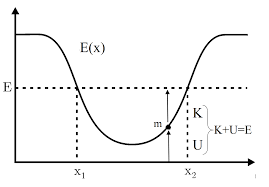The image above represents potential energy.

To compute for the potential energy, three essential parameters are needed and these parameters are mass (m), height (h) and acceleration due to gravity (g).

The formula for calculating the potential energy:

P.E = mgh

Where;
P.E. = Potential Energy
m = Mass
g = acceleration due to gravity
h = Height

Let’s solve an example;
Find the potential energy when the mass is 12 with a height of 24 and acceleration due to gravity of 9.8.

This implies that;
m = Mass = 12
g = acceleration due to gravity = 9.8
h = Height = 24

P.E = mgh
P.E = 12 x 9.8 x 24
P.E = 2822.4

Therefore, the potential energy is 2822.4 Joules (J).

Calculating the Mass when Potential Energy, Height and Acceleration due to Gravity is Given.

m = P.E / gh

Where;
m = Mass
P.E. = Potential Energy
g = acceleration due to gravity
h = Height

Let’s solve an example;
Find the Mass when potential energy is 450 with a height of 30 and acceleration due to gravity of 10.

This implies that;
P.E. = Potential Energy = 450
g = acceleration due to gravity = 10
h = Height = 30

m = P.E / gh
m = 450 / 10 x 30
m = 450 / 300
m = 1.5

Therefore, the mass is 1.5.

Calculating the Height when Potential Energy, Mass and Acceleration due to Gravity.

h = P.E / mg

Where;
h = Height
m = Mass
P.E. = Potential Energy
g = acceleration due to gravity

Let’s solve an example;
Find the Height when potential energy is 500 with a mass of 25 and acceleration due to gravity of 10.2.

This implies that;
P.E. = Potential Energy = 500
g = acceleration due to gravity = 10.2
m = Mass = 25

h = P.E / mg
h = 500 / 25 x 10.2
h = 500 / 255
h = 1.96

Therefore, the height is 1.96.

Nickzom Calculator – The Calculator Encyclopedia is capable of calculating the potential energy.

To get the answer and workings of the potential energy using the Nickzom Calculator – The Calculator Encyclopedia. First, you need to obtain the app.

You can get this app via any of these means:

To get access to the professional version via web, you need to register and subscribe for NGN 1,500 per annum to have utter access to all functionalities.
You can also try the demo version via https://www.nickzom.org/calculator

Once, you have obtained the calculator encyclopedia app, proceed to the Calculator Map, then click on Work, Energy and Time under Physics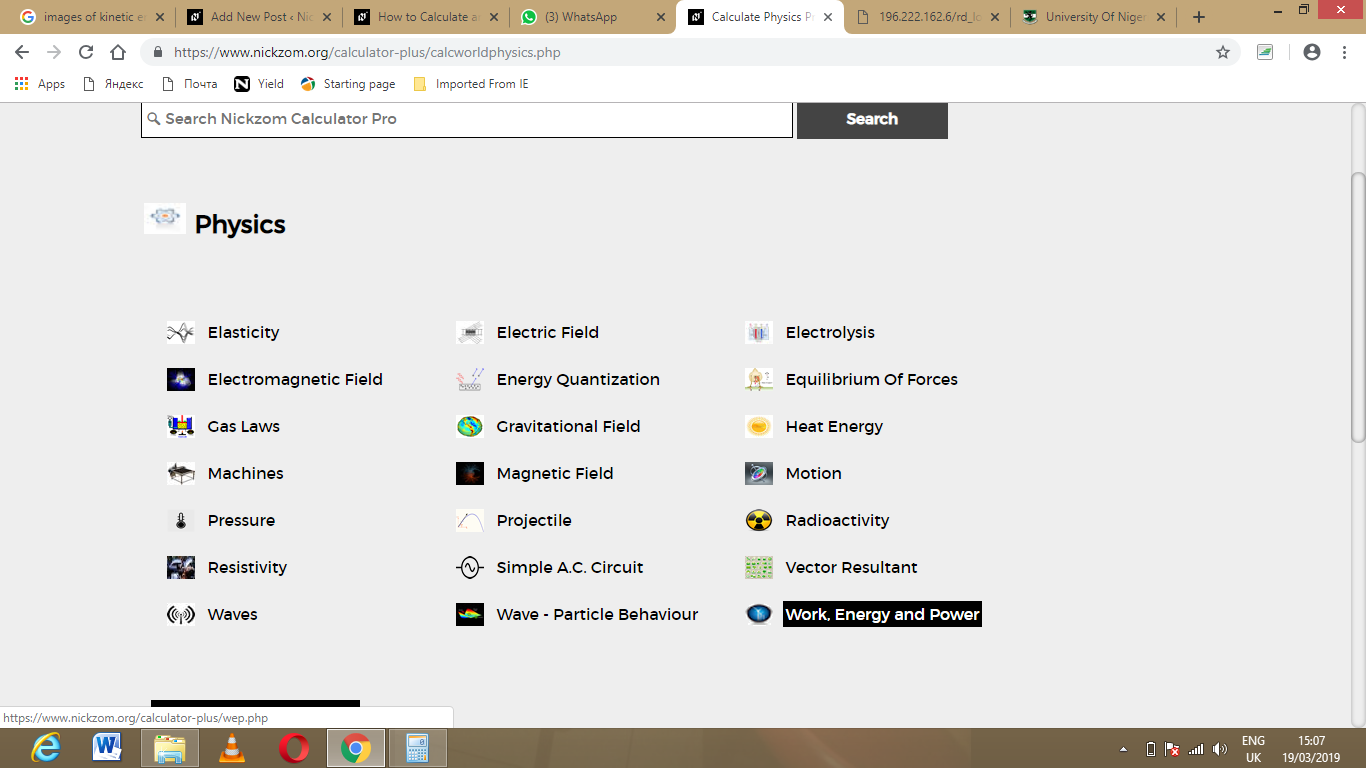Now, Click on Potential energy under Work, Energy and Time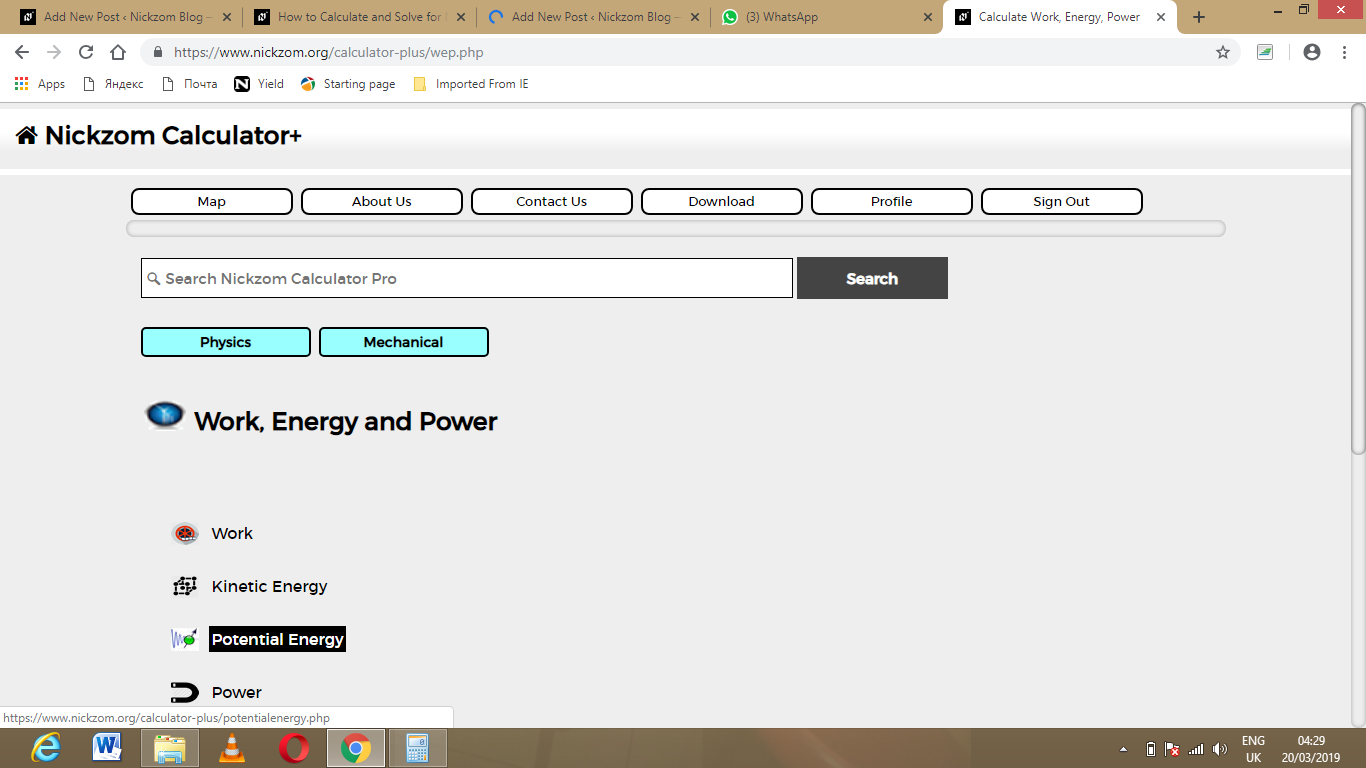The screenshot below displays the page or activity to enter your values, to get the answer for the potential energy according to the respective parameters which are the mass (m), height (h) and acceleration due to gravity (g).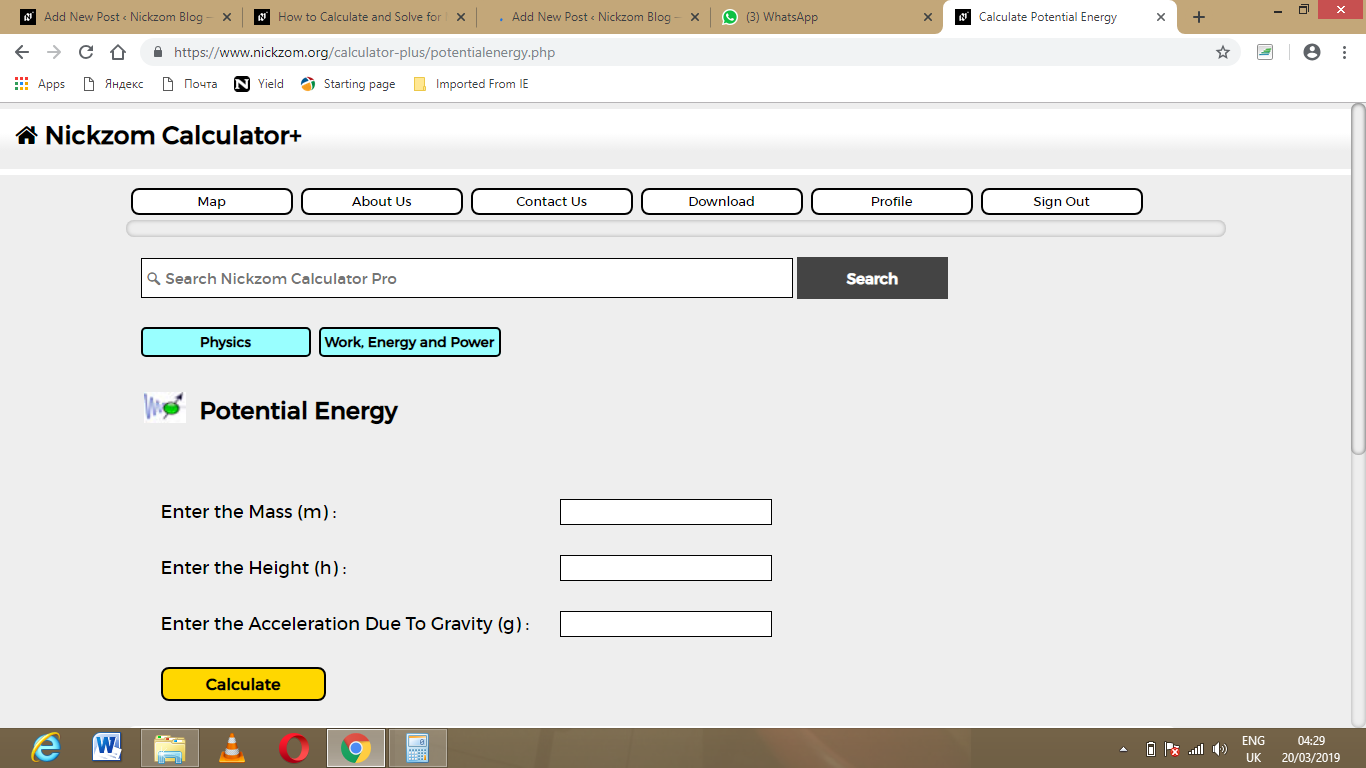Now, enter the values appropriately and accordingly for the parameters as required by the mass (m) is 12, height (h) is 24 and acceleration due to gravity (g) is 9.8.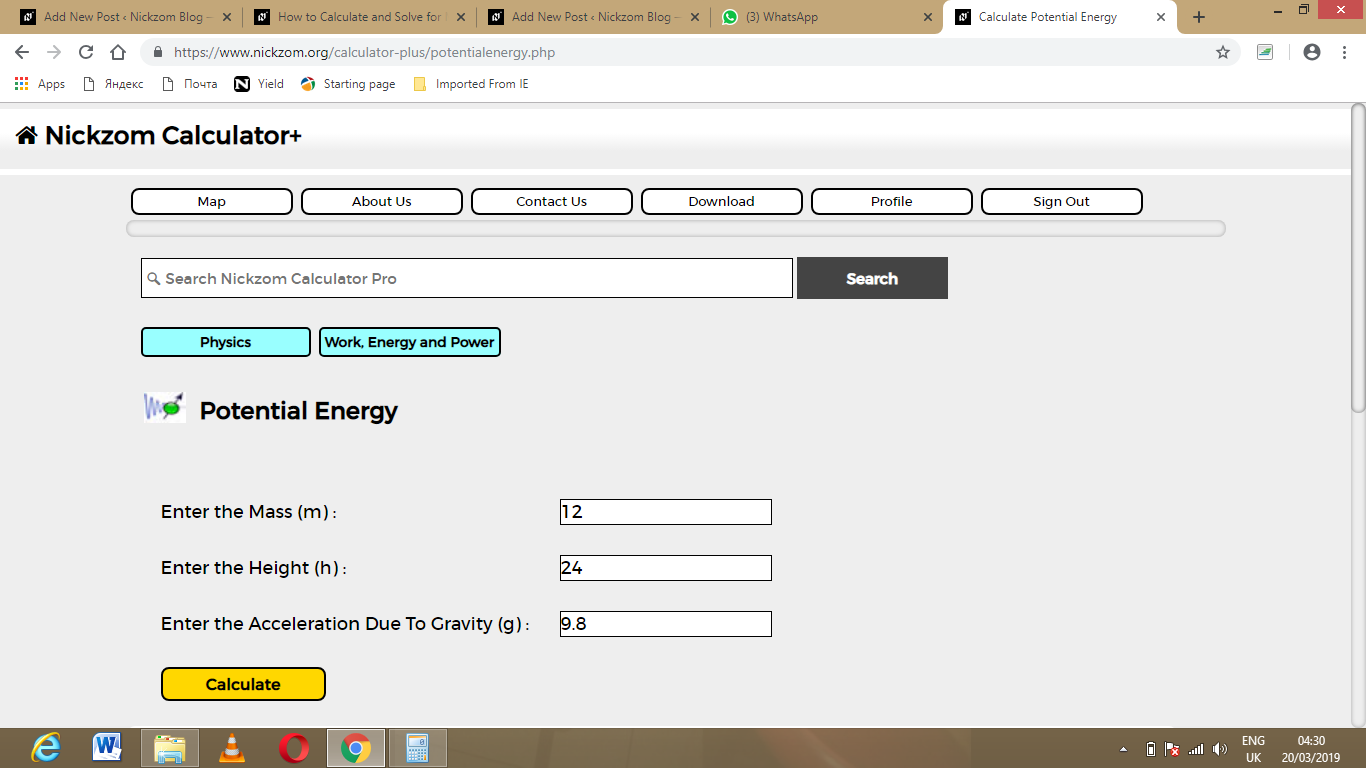Finally, Click on Calculate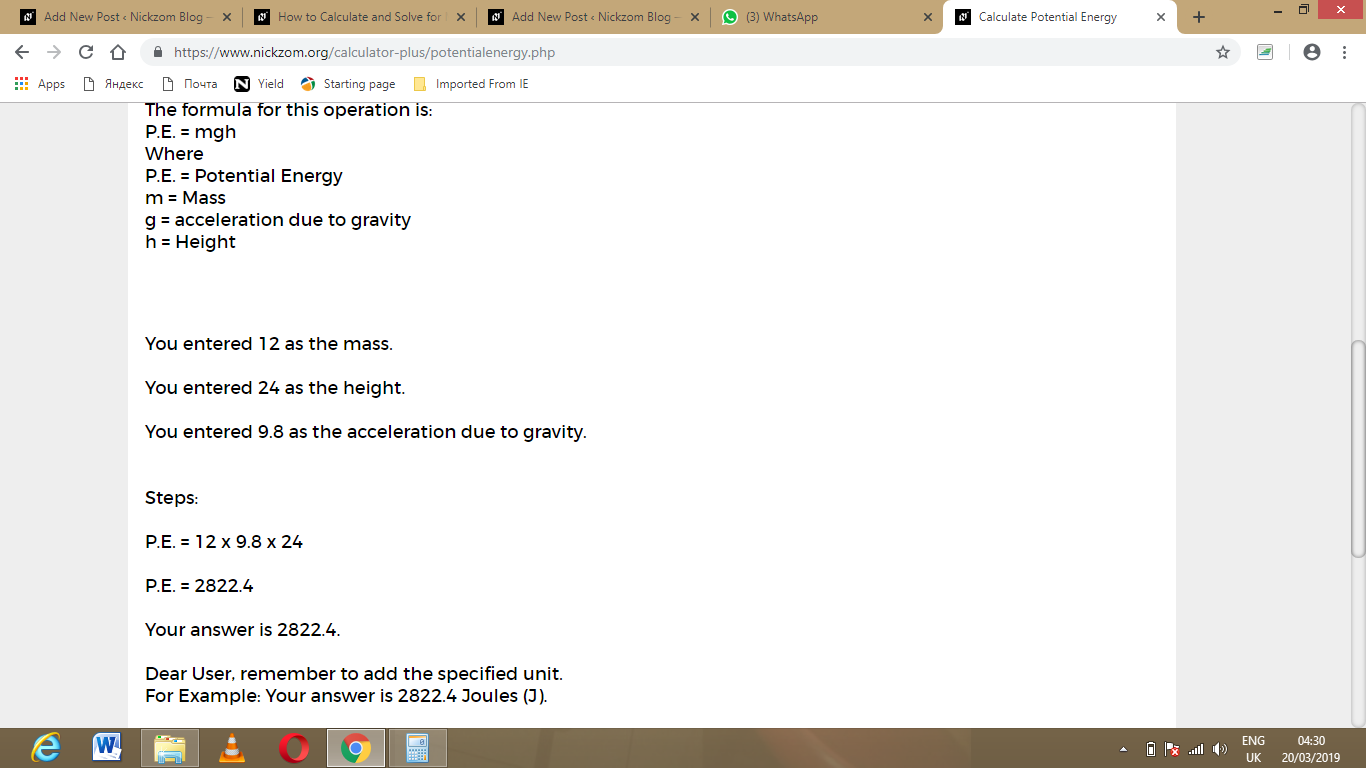As you can see from the screenshot above, Nickzom Calculator – The Calculator Encyclopedia solves for the potential energy and presents the formula, workings and steps too.